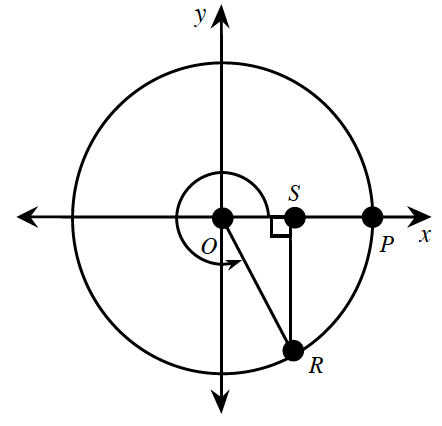### Home > INT3 > Chapter 9 > Lesson 9.1.4 > Problem9-46

9-46.

The measure of $∠ROS$ in $ΔROS$ below is $60^\circ$.1. The curved arrow represents the rotation of $\overline { O R }$, beginning from the positive $x$-axis. Through how many degrees has $\overline { O R }$ rotated?

Remember that a whole circle is $360^\circ$.

2. If $OR = 1$, what are the exact length of $OS$ and $SR$?

Remember the $30^\circ-60^\circ-90^\circ$ triangle.

3. What are the exact coordinates of point $R$?

$\left(\text{cos}(300^{^{\circ}}), \text{sin}(300^{^{\circ}})\right)\text{ or }\left(\frac{1}{2}, - \frac{\sqrt{3}}{2}\right)$